Скачать презентацию Supplement to Chapter 4 Interest Rate Conversions Definitions

ecae0f1373de5458024952f5bd32bfdd.ppt

• Количество слайдов: 20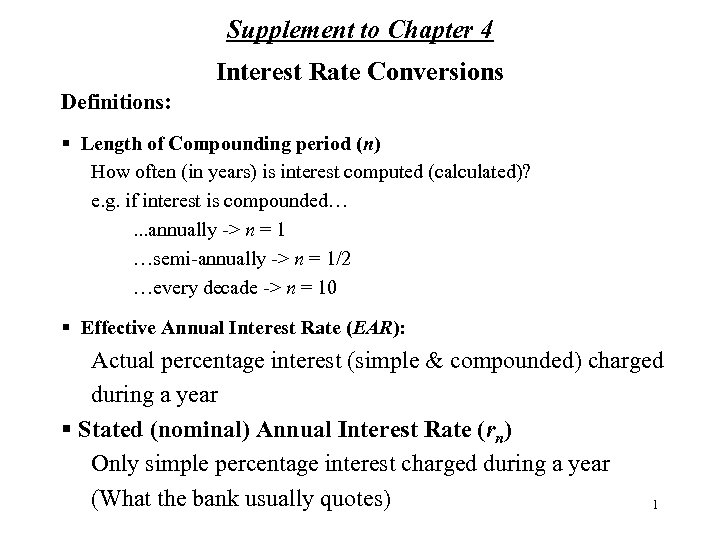Supplement to Chapter 4 Interest Rate Conversions Definitions: § Length of Compounding period (n) How often (in years) is interest computed (calculated)? e. g. if interest is compounded…. . . annually -> n = 1 …semi-annually -> n = 1/2 …every decade -> n = 10 § Effective Annual Interest Rate (EAR): Actual percentage interest (simple & compounded) charged during a year § Stated (nominal) Annual Interest Rate (rn) Only simple percentage interest charged during a year (What the bank usually quotes) 1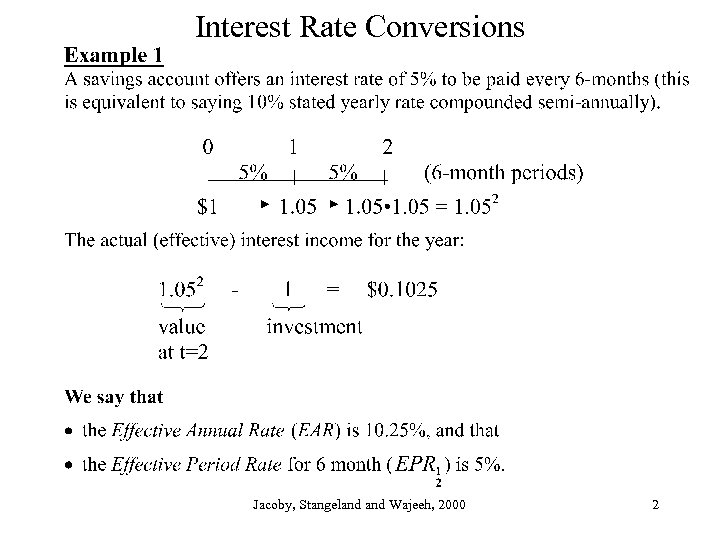Interest Rate Conversions Jacoby, Stangeland Wajeeh, 2000 2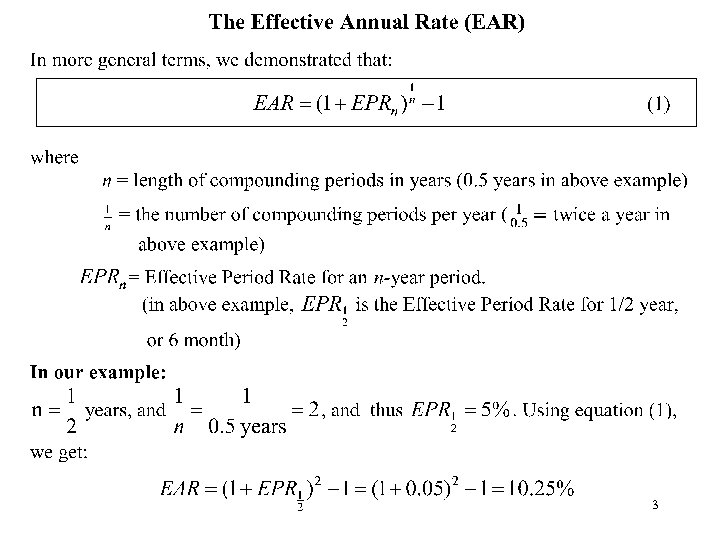3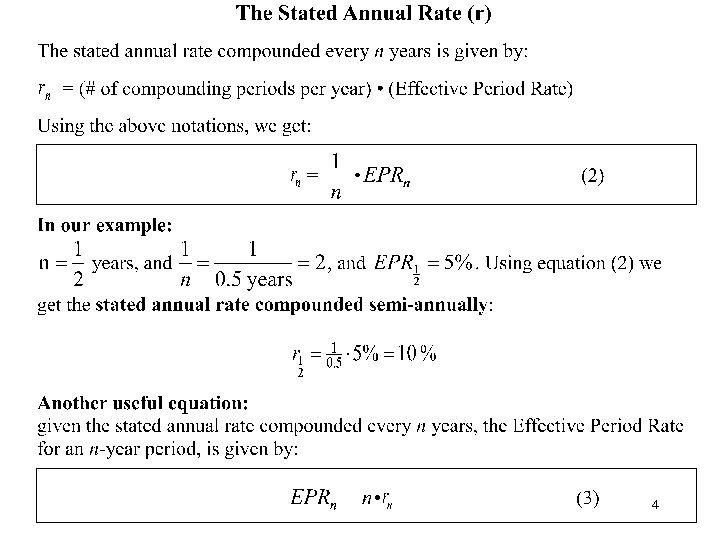4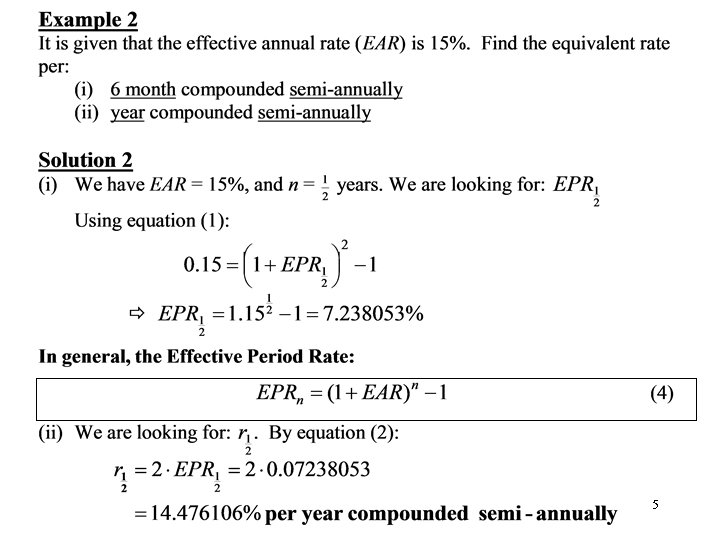5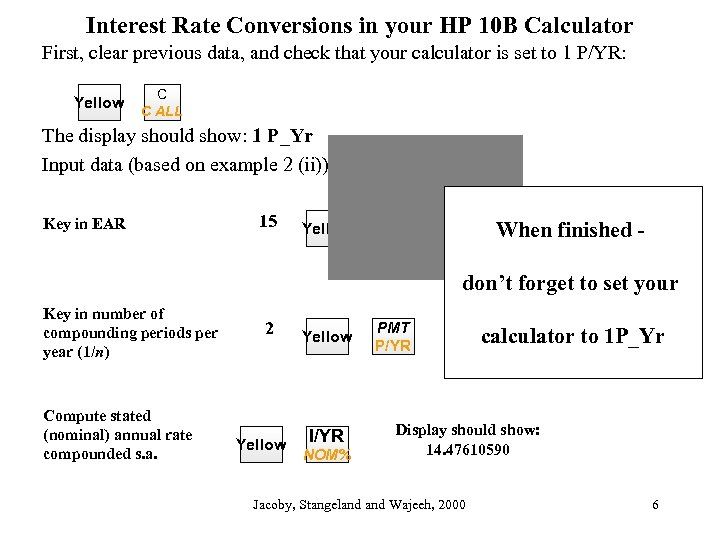Interest Rate Conversions in your HP 10 B Calculator First, clear previous data, and check that your calculator is set to 1 P/YR: Yellow C C ALL The display should show: 1 P_Yr Input data (based on example 2 (ii)): Key in EAR 15 Yellow PV When finished - EFF% don’t forget to set your Key in number of compounding periods per year (1/n) Compute stated (nominal) annual rate compounded s. a. 2 Yellow I/YR NOM% PMT P/YR calculator to 1 P_Yr Display should show: 14. 47610590 Jacoby, Stangeland Wajeeh, 2000 6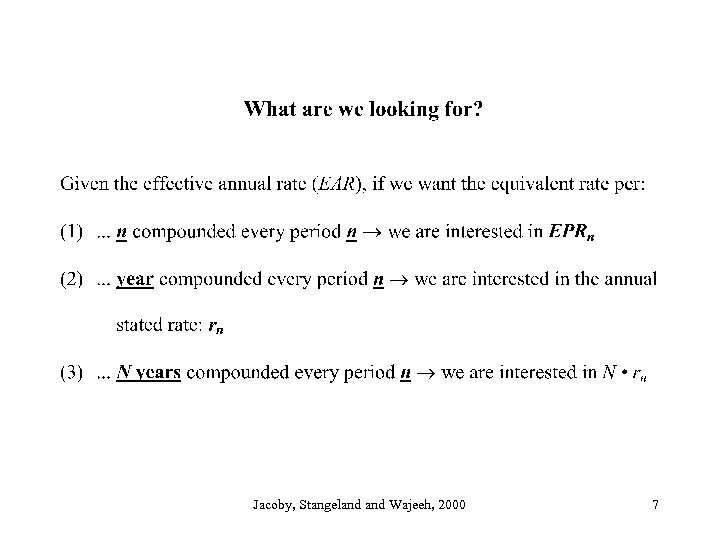Jacoby, Stangeland Wajeeh, 2000 7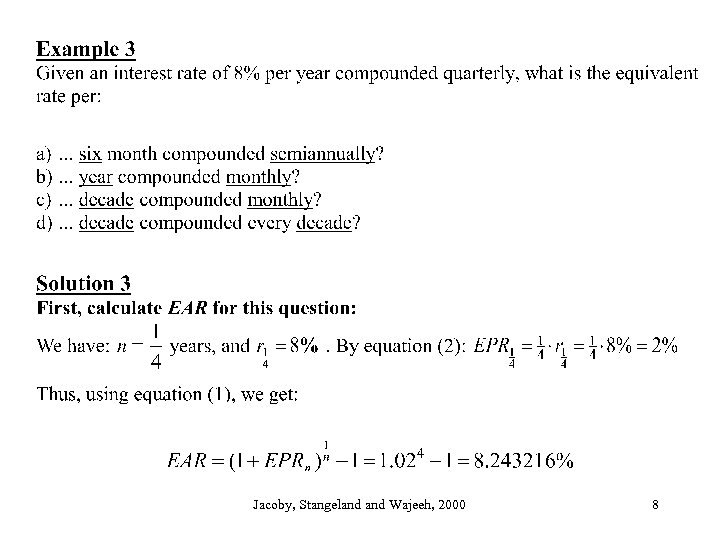Jacoby, Stangeland Wajeeh, 2000 8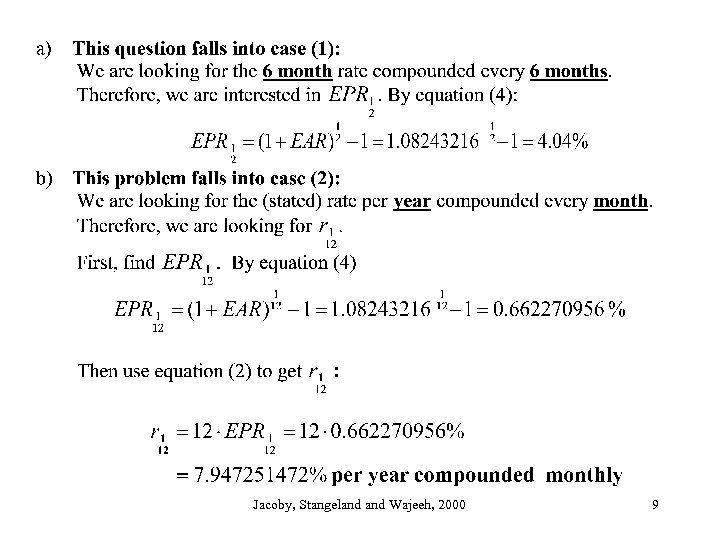Jacoby, Stangeland Wajeeh, 2000 9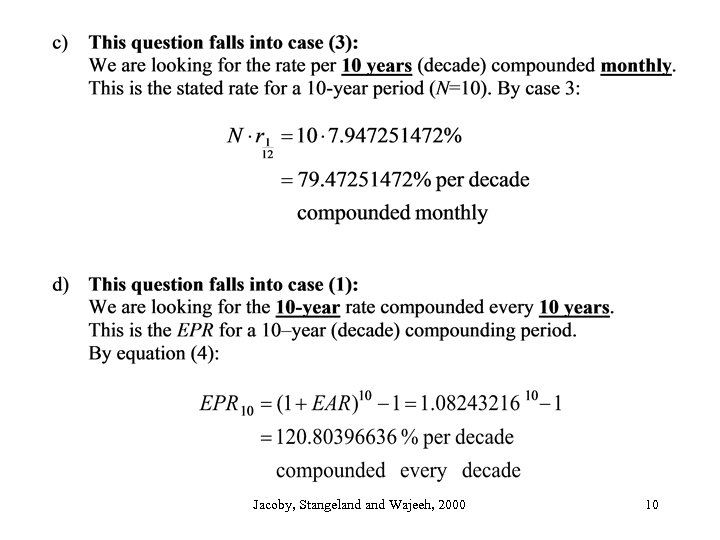Jacoby, Stangeland Wajeeh, 2000 10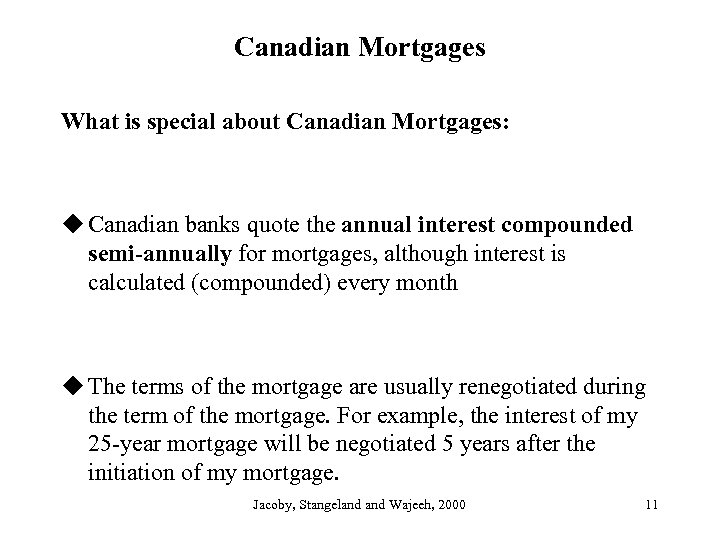Canadian Mortgages What is special about Canadian Mortgages: u Canadian banks quote the annual interest compounded semi-annually for mortgages, although interest is calculated (compounded) every month u The terms of the mortgage are usually renegotiated during the term of the mortgage. For example, the interest of my 25 -year mortgage will be negotiated 5 years after the initiation of my mortgage. Jacoby, Stangeland Wajeeh, 2000 11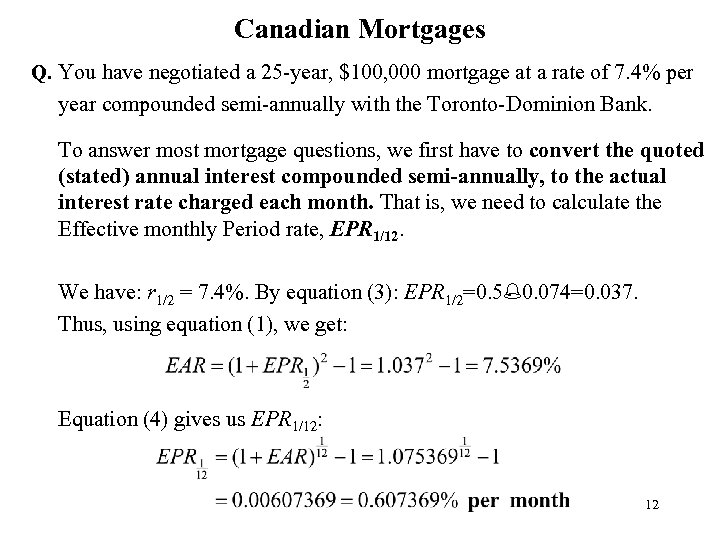Canadian Mortgages Q. You have negotiated a 25 -year, \$100, 000 mortgage at a rate of 7. 4% per year compounded semi-annually with the Toronto-Dominion Bank. To answer most mortgage questions, we first have to convert the quoted (stated) annual interest compounded semi-annually, to the actual interest rate charged each month. That is, we need to calculate the Effective monthly Period rate, EPR 1/12. We have: r 1/2 = 7. 4%. By equation (3): EPR 1/2=0. 5%0. 074=0. 037. Thus, using equation (1), we get: Equation (4) gives us EPR 1/12: 12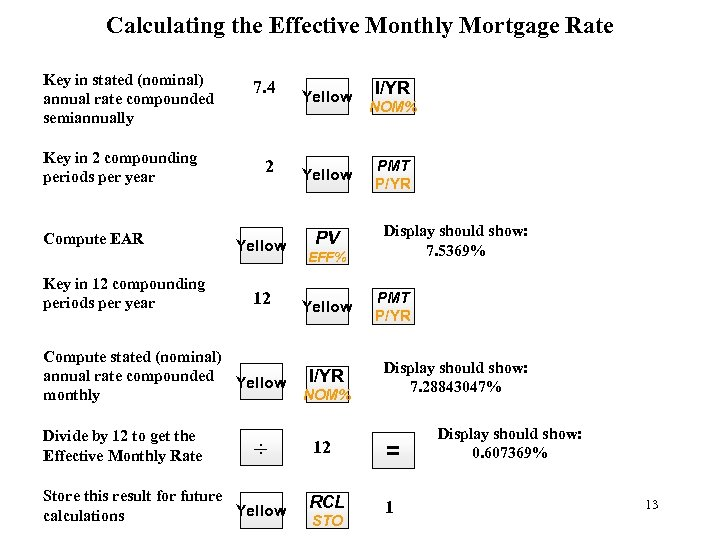Calculating the Effective Monthly Mortgage Rate Key in stated (nominal) annual rate compounded semiannually Key in 2 compounding periods per year Compute EAR Key in 12 compounding periods per year 7. 4 2 Yellow 12 Compute stated (nominal) annual rate compounded Yellow monthly Divide by 12 to get the Effective Monthly Rate ÷ Store this result for future Yellow calculations Yellow PV EFF% Yellow I/YR NOM% PMT P/YR Display should show: 7. 5369% PMT P/YR Display should show: 7. 28843047% 12 = RCL 1 STO Display should show: 0. 607369% 13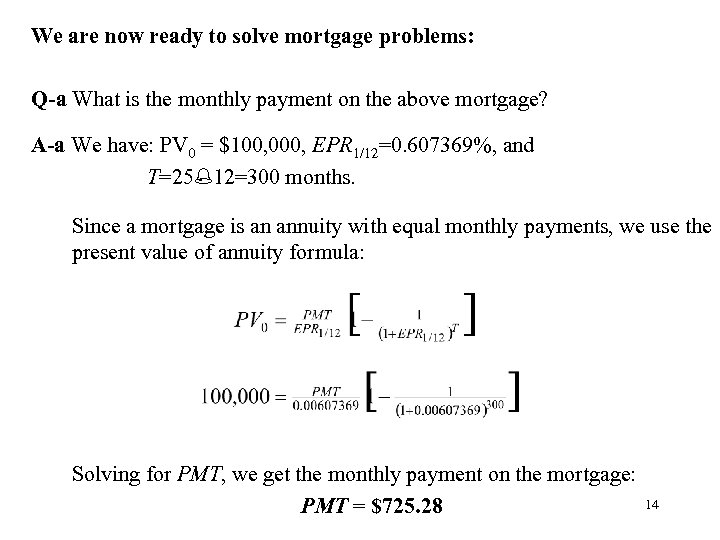We are now ready to solve mortgage problems: Q-a What is the monthly payment on the above mortgage? A-a We have: PV 0 = \$100, 000, EPR 1/12=0. 607369%, and T=25%12=300 months. Since a mortgage is an annuity with equal monthly payments, we use the present value of annuity formula: Solving for PMT, we get the monthly payment on the mortgage: PMT = \$725. 28 14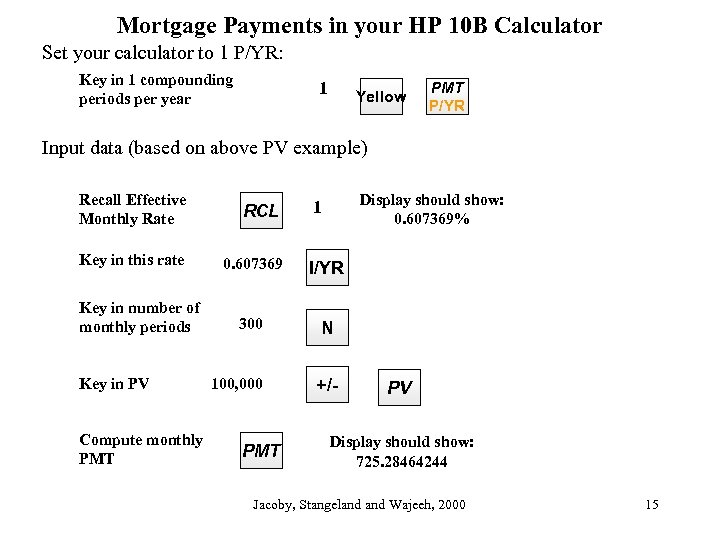Mortgage Payments in your HP 10 B Calculator Set your calculator to 1 P/YR: Key in 1 compounding periods per year 1 Yellow PMT P/YR Input data (based on above PV example) Recall Effective Monthly Rate RCL Key in this rate 0. 607369 I/YR 300 N 100, 000 +/- Key in number of monthly periods Key in PV Compute monthly PMT Display should show: 0. 607369% 1 PV Display should show: 725. 28464244 Jacoby, Stangeland Wajeeh, 2000 15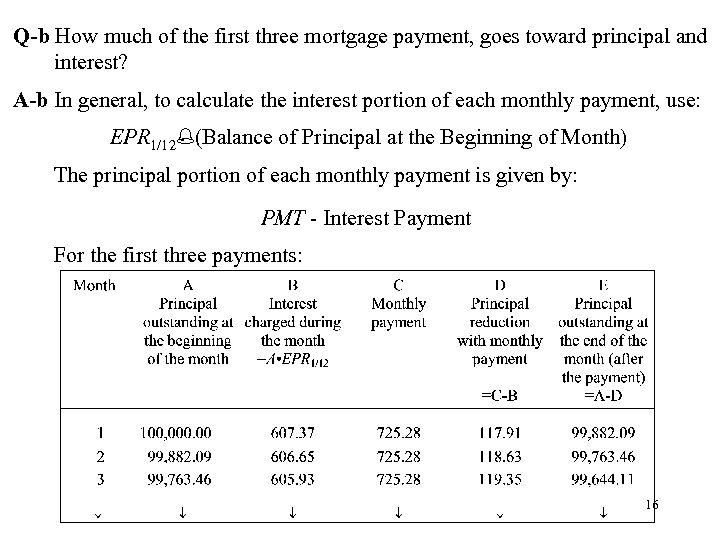Q-b How much of the first three mortgage payment, goes toward principal and interest? A-b In general, to calculate the interest portion of each monthly payment, use: EPR 1/12%(Balance of Principal at the Beginning of Month) The principal portion of each monthly payment is given by: PMT - Interest Payment For the first three payments: 16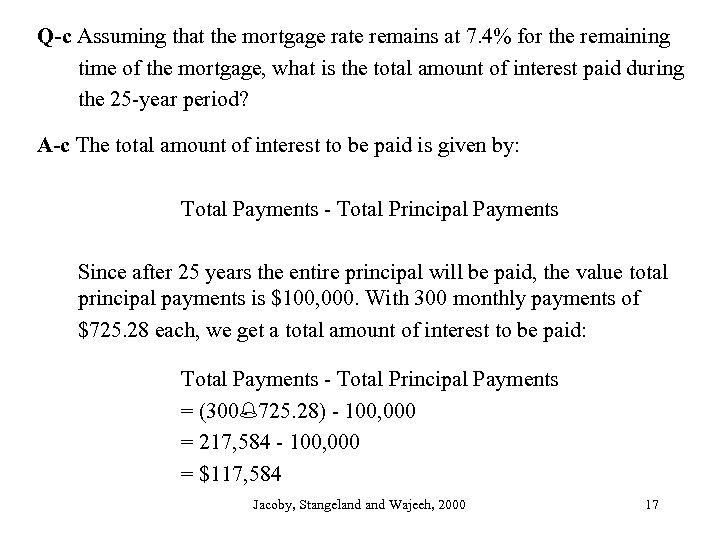Q-c Assuming that the mortgage rate remains at 7. 4% for the remaining time of the mortgage, what is the total amount of interest paid during the 25 -year period? A-c The total amount of interest to be paid is given by: Total Payments - Total Principal Payments Since after 25 years the entire principal will be paid, the value total principal payments is \$100, 000. With 300 monthly payments of \$725. 28 each, we get a total amount of interest to be paid: Total Payments - Total Principal Payments = (300%725. 28) - 100, 000 = 217, 584 - 100, 000 = \$117, 584 Jacoby, Stangeland Wajeeh, 2000 17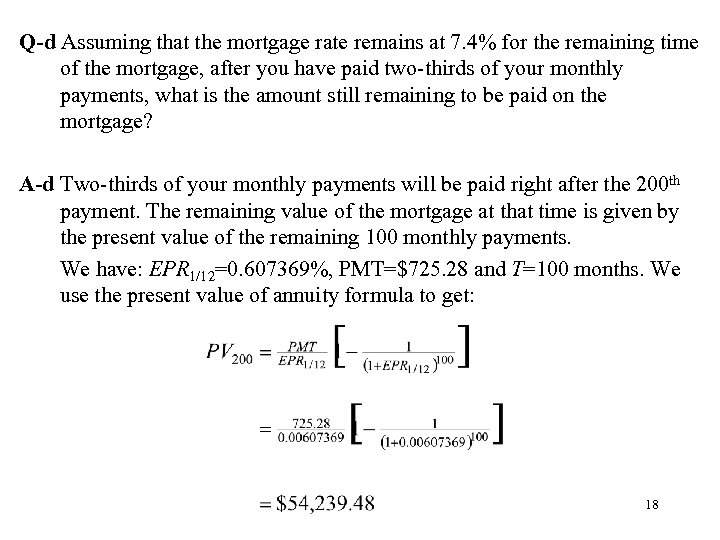Q-d Assuming that the mortgage rate remains at 7. 4% for the remaining time of the mortgage, after you have paid two-thirds of your monthly payments, what is the amount still remaining to be paid on the mortgage? A-d Two-thirds of your monthly payments will be paid right after the 200 th payment. The remaining value of the mortgage at that time is given by the present value of the remaining 100 monthly payments. We have: EPR 1/12=0. 607369%, PMT=\$725. 28 and T=100 months. We use the present value of annuity formula to get: 18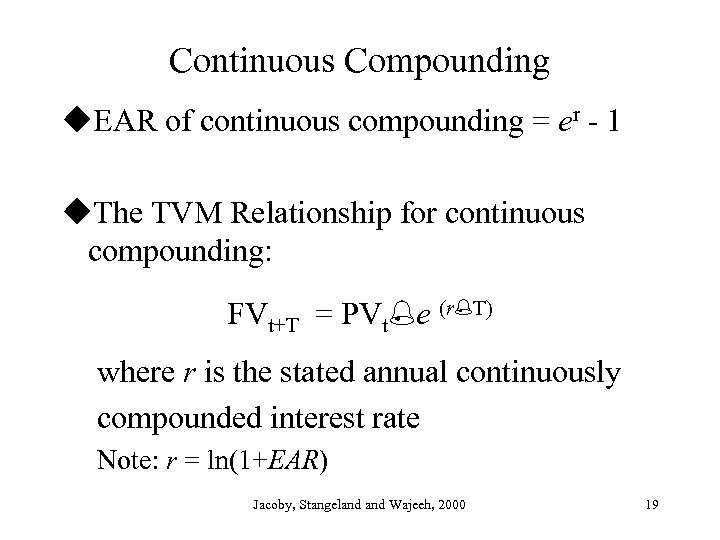Continuous Compounding u. EAR of continuous compounding = er - 1 u. The TVM Relationship for continuous compounding: FVt+T = PVt%e (r%T) where r is the stated annual continuously compounded interest rate Note: r = ln(1+EAR) Jacoby, Stangeland Wajeeh, 2000 19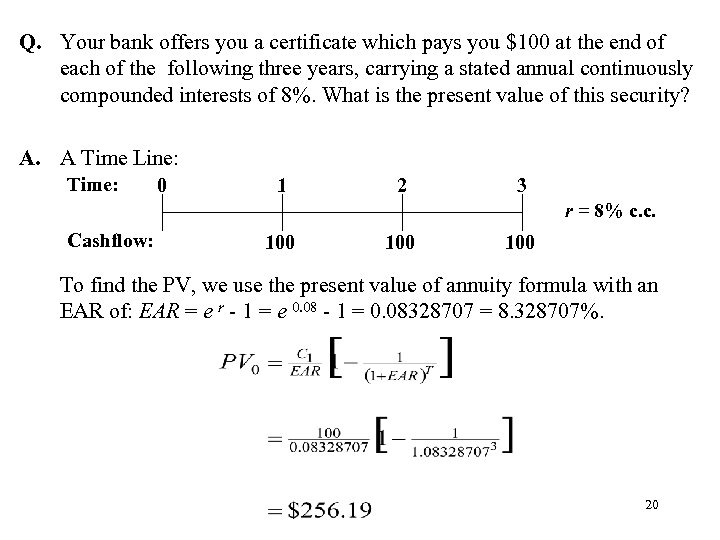Q. Your bank offers you a certificate which pays you \$100 at the end of each of the following three years, carrying a stated annual continuously compounded interests of 8%. What is the present value of this security? A. A Time Line: Time: 0 1 2 3 r = 8% c. c. Cashflow: 100 100 To find the PV, we use the present value of annuity formula with an EAR of: EAR = e r - 1 = e 0. 08 - 1 = 0. 08328707 = 8. 328707%. 20